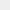# BRSA: January – April total profit of banks at 98.2 billion TRY

## According to the monthly data of the BRSA; While the total profit of Turkish banks was 98.2 billion liras in the January-April period, it was 20.7 billion liras in the same period of last year.

6544 kez okundu.According to the monthly data of the BRSA; While the total profit of Turkish banks was 98.2 billion liras in the January-April period, it was 20.7 billion liras in the same period of last year.

·        Ave. NPL ratio was 2.75%, versus 3.74% last year.

·        Ave. standard capital adequacy ratio was 20.4% versus 18% last year.

·        Ave. core capital adequacy ratio was 16% versus 14% last year.

·        Total assets amounted to TRY 10.4 trillion versus TRY 6.53 trillion last year.

The Turkish banking sector experienced a rapid increase in profits in April. The profit of the sector increased by 707.8% in April compared to the same period of the previous year and became 34.9 billion TRY. On the asset side, the increase in the difference between the domestic funding costs of banks and the interest rates of loans and the increase in inflation-indexed bonds led to an increase in the sector's profit. Despite the continued increase in inflation in the second quarter of the year and the deterioration in economic activity due to the high profit margin, the positive outlook in the sector's profit seems likely to continue.

Despite the increase in NPL, the NPL ratio of loans decreased due to the stronger increase in loans and realized as 2.75% in April, the lowest level since August 2018. The increase in the annual rate of increase in NPLs, which started in 4Q21, continued in the first part of 2022, with the end of the BRSA's practice of increasing the waiting period for the transfer of overdue loans to non-performing loans from 90 days to 180 days.

With the falling interest rates, the share of loans in total assets increased. While the annual rate of increase in loans was 37% at the end of 2021, it increased to 48.5% in April 2022 due to the increase in the exchange rate and high demand for borrowing in an environment of high inflation. The determinants of the rapid increase in loans in 2021 were private banks. It is seen that private banks are again the determining factor in the slowdown in the loan growth rate due to the base effect created. The increase in loans also resulted in an increase in net fee and commission income.

Until 4Q21, the annual growth rate of total assets slowed down due to the slowdown in the annual growth rate of both securities and loans. It is observed that the annual rate of increase in total assets increased with the annual increase in securities and loans, which started in 4Q21 and continued in 1Q22. In April 2022, the annual growth rate of the FX securities portfolio, which constituted 51.4% of the total securities, reached 83% due to the base effect as well as the increase in the exchange rate and the value of the inflation-indexed securities.

Despite the decrease in the annual growth rate of FX deposits, which constitute 56.6% of the total deposits, the annual growth rate of TRY deposits increased from 21.6% at the end of 2021 to 61.2% in April. The annual growth rate of FX deposits decreased from 79.3% to 70% between the same periods. The annual rate of increase in total deposits increased from 53.5% to 66.1%. The increase in the tendency to deposits due to the increase in the exchange rate and the exchange rate protected deposit accounts caused an increase in the share of deposits. With the effect of the rapid increase in deposits, the rate of conversion of deposits to loans was 97.1% in April.

In April 2022, deposits were concentrated in time deposits. In April, where demand deposits constituted 37.2% of total deposits, the share of 1-3 month deposits was 33%. Until 4Q21, deposits were concentrated in 1-3 month time deposits. However, this situation has changed due to the increase in the exchange rate and the effect of foreign exchange-protected deposit accounts. While the increase in the exchange rate led to an increase in demand deposits, opening FX-protected deposit accounts with a maturity of at least 3 months caused an increase in deposits with a maturity of more than 3 months.

The decrease in the share of non-deposit resources resulted from the decrease in the share of loans to banks and funds provided from repo transactions. Due to the decrease in the annual growth rate of both TL and FX non-deposit resources, the annual growth rate in total non-deposit resources decreased from 55.9% at the end of 2021 to 48.2% in April 2022.

The capital adequacy ratio (CAR), which shows the ratio of the sector's capital to risk-weighted assets, which was 18% at the end of 2021, became 20% in April 2022. In addition to the base effect, there was a recovery in non-interest incomes throughout 2021 with the easing of the pandemic. It is observed that the annual rate of increase in non-interest incomes was 56.7% in April 2022.

Kaynak Tera Yatırım
Hibya Haber Ajansı

Yorum YapKalan karakter sayısı : 500